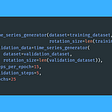# Conv1D Layers in Time-Series

For this blog, I will describe the parameters of the Conv1D layer and a simple WaveNet-like DCNN(Dilated Convoluted Neural Network) used for a time-series problem. Hackathon contestants predominantly solved time-series problems with ARIMA and GRU or LSTM neural networks. However, recent DCNN architectures have been showing up on winning hackathon notebooks. A convolutional layer is a piece of a neural network architecture often used for image classification. Still, CNN can also be applied as a sequence model with the right formatting and parameterization.

The Conv1D layer has some interesting characteristics that I can use to help solve sequence problem. I came across an exciting architecture from a blog post created by Joseph Eddy, which explains a build-up of a simple WaveNet-like model for time series. Check the blog out here.

The objective is to use past observations to predict the next time step. Separating the dataset into windows of input and output is necessary before fitting the model. The diagram below represents an input shape of 8 and an output shape of 1. The objective is to use the inputs(past observations) to predict the output(future).

The inputs from the diagram above take in the 8 inputs to output the next time step. The model can get more involved by adding dense layers between the input and the output. However, it might be even more interesting to create dense layers where the inputs are more refined. For example, the first hidden layer’s output might take in the 2 time-separated inputs in a binary tree-like fashion. I can use the Conv1D layer as a time separated dense layer for this task with 6 hidden layers.

The above architecture can become problematic as the input size increases because the number of hidden layers will increase one to one. If the input shape were 16 instead of 8, the number of hidden layers would increase by 8.

To reduce the number of hidden layers, a WaveNet architecture used dilated convolutions, which practically skips some of the inputs in between the hidden layers. Instead of applying the filter(weights) in a sequential form, the information between the hidden layers skips at a “dilation rate.”

A sample neural network might look like the one below. The for loop is only to collect the layers of the skipped inputs as the dilation rates increase.

## Filters

The filter parameter is the number of filters/weights applied to the n_inputs and n_features shape. For the above example, with an input shape of 8, 1(8 inputs, 1 feature), the output of Conv1D(filters = 16) will produce 16 different outcomes resulting in a shape of (8, 16).

## Kernel Size

The kernel size is the size of the sequential window of the input. If the kernel size is set at 1, then each time interval will have its kernel and therefore, the output shape won’t change from the (8, 16)[16 filters as above example]. However, a kernel size of 2 means that the window of input is the 2 adjacent inputs like below.

Without padding, the output of the Conv1D layer with kernel size of 2 will reduce to shape from (8, 16) to (7,16).

With ‘causal’ padding, zeros are included only on the left side of the time input so that the output can match the original input shape(8).

Setting ‘same’ under the padding parameter will fill in zeros from both left and right sides of the input shape. It’s unnecessary to define this when kernel size is at 1, but I included it in the code for the code example.

## Dilation Rate

The dilation rate is the skipping rate for the kernels. At dilation_rate = 1 and a kernel_size = 2, the inputs are paired sequentially. At dilation_rate = 2, the inputs from the first hidden layer(dilation_rate=1) will skip 1 as shown below. I don’t believe the greyed neurons appear during computation; however, I included them for this visualization.

--

--

--

## More from Albert Um

Hello! My name is Albert Um.

Love podcasts or audiobooks? Learn on the go with our new app.

## Image Classification with Logistic Regression on American Sign Language (MNIST Dataset)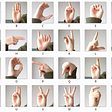## Steps in NLP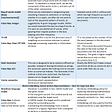## Understanding Machine Learning through Memes## Grant Access using Machine Learning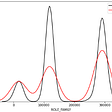## Building footprint comparison in Cambodia: Orbital Insight and OpenStreetMap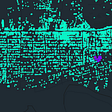## 5 Application of machine learning in customer analytics## Attention Mechanisms in Vision Models## Food Wars## Albert Um

Hello! My name is Albert Um.

## A Deep Learning Based Hybrid Approach for Short-Term Forecasting of spread of COVID-19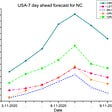## Deep-learning model SCINet applied to crypto-market time-series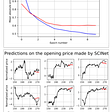## Prepare Time Series Data For Time Series Forecasting With Deep Learning — Part 2